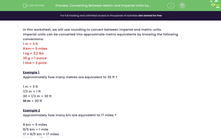# Convert Between Metric and Imperial Units

In this worksheet, students will convert between metric and imperial units.Key stage:  KS 3

Curriculum topic:   Number

Curriculum subtopic:   Round/Approximate Numbers Appropriately

Popular topics:   Rounding worksheets

Difficulty level:#### Worksheet Overview

In this activity, we will convert between imperial and metric units.

Imperial units can be converted into approximate metric equivalents by knowing the following conversions:

1 m = 3 ft
8 km = 5 miles
1 kg = 2.2 lbs
30 g = 1 ounce
1 litre = 2 pints

Example 1

Approximately how many metres are equivalent to 30 ft ?

1 m = 3 ft

3 ft = 1 m

3 x 10 = 30 ft, so 1 x 10 = 10 m

30 ft = 10 m

10 m = 30 ft

Example 2

Approximately how many km are equivalent to 17 miles ?

8 km = 5 miles

8/5 km = 1 mile

8/5 km x 17 = 17 miles

27.2 km = 17 miles

How do you feel about these conversions now? Are you ready to have a go at some questions?

You can check back to these conversion charts at any point by clicking on the pink button at the side of the screen.### What is EdPlace?

We're your National Curriculum aligned online education content provider helping each child succeed in English, maths and science from year 1 to GCSE. With an EdPlace account you’ll be able to track and measure progress, helping each child achieve their best. We build confidence and attainment by personalising each child’s learning at a level that suits them.

Get started